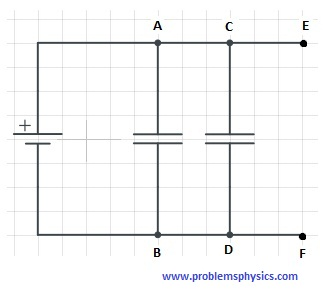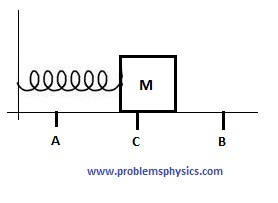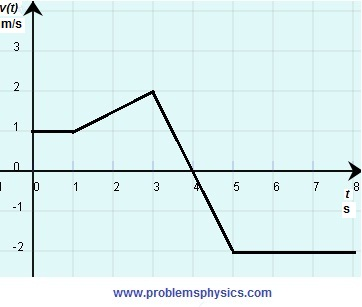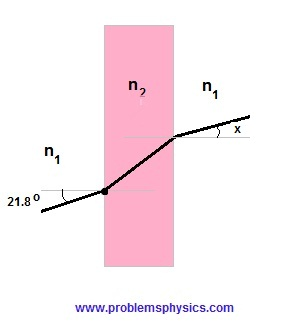# Free SAT II Physics Practice Questions with Solutions Sample 1

 Sat Physics subject questions, with answers, similar to the questions in the SAT test are presented. The answers are at the bottom of the page and the detailed solutions are also included. A particle moves 80 cm South in 0.12 seconds and then 60 cm West in 0.08 seconds. What is the magnitude of the average velocity of the particle? A) 700 cm/s B) 1417 cm/s C) 500 cm/s D) 250 cm/s E) 709 cm/s When a car's speed changes from 20 m/s to 40 m/s, its kinetic energy A) stays the same B) is doubled C) is halved D) is tripled E) is quadrupled The graph below is that of the velocity of a moving particle. At which of the points A, B, C, D or E is the acceleration highest?A) A B) B C) C D) D E) E The graph below is that of the height of a ball thrown vertically upward. At which point is the velocity close or equal to zero?A) A B) B C) C D) D E) E The capacitors between A and B and C and D are equivalent and have capacitance C each. How many equivalent capacitors, with capacitance C, we need to put in series between E and F so that the total capacitance in the circuit is equal to 2.5C?A) 2 B) 3 C) 4 D) 5 E) 6 All resistors in the circuit below are equivalent. What is the ratio of the voltage drop between points A and B and the voltage drop between points B and C?A) 1/3 B) 1/9 C) 1/6 D) 1/2 E) 1 Vectors u and v have magnitudes of 15 and 7 units respectively. What is the possible highest value of the magnitude of the vector 3u - 2v? A) 31 units B) 45 units C) 66 units D) 59 units E) 8 units In order to increase the frequency of oscillations, for small amplitudes, of a pendulum of length L with a bob of mass M, you need to A) increase L B) decrease L C) increase M D) decrease M E) decrease both M and L What is the tension in the upper string of the system made up of two blocks of metal each of mass 5 kg, suspended from the ceiling by strings of negligible mass, as shown above.A) 49 N B) 25 N C) 980 N D) 98 N E) 10 N In the figure below, is shown mass M attached to a spring. The initial position of mass M is at point C. A and B are the extreme positions of the mass as it oscillates. The kinetic energy of mass M is maximum atA) point A only B) point B only C) point C only D) points A and B E) points C and B What is the y-component of the resultant force when 3 forces, whose magnitudes are given, are acting on an object at point O as shown in the figure?A) 12 cos(α) + 20 sin(θ) B) 12 sin(α) + 20 sin(θ) C) -10 D) 12 sin(α) + 20 sin(θ) - 10 E) 12 cos(α) + 20 sin(θ) - 10 The graph of the velocity against the time of a moving object is shown below. What is the displacement between t = 3 and t = 5?A) 4 m B) 2 m C) equal or close to zero D) -4 m E) -2 m Three particles with charges +q at points A, -q at point B and +q at point C, are located at vertices of an equilateral triangle and the line through A and B is parallel to the x axis. What is true about the resultant force acting on the charge at point C?A) its x and y components are equal B) its y component is equal to zero C) its x component is equal to zero D) its x and y components are equal to zero E) its direction makes an angle of 45 degrees with the x axis What is the momentum of a moving object that has a mass of 100 Kg and kinetic energy of 20,000 Joules? A) 10,000 Kg.m/s B) 100 Kg.m/s C) 1000 Kg.m/s D) 2000,000 Kg.m/s E) 2000 Kg.m/s Ball $A$ of mass $m$ and velocity $v$ collides with ball $B$ of mass m and velocity $-v$. What are the velocities $V_A$ and $V_B$ of the two balls after collision? A) $V_A=-v$ and $V_B=v$ B) $V_A=v$ and $V_B=-v$ C) $V_A=-v$ and $V_B=-v$ D) $V_A=v$ and $V_B=v$ E) $V_A=0$ and $V_B=v$ The distance between two successive crests of a wave is $10^{-12}$ meters. The time between the arrival of successive crests is equal to $10^{-11}$ seconds. What is the speed of the wave? A) 100 m B) 0.1 mm C) 10 cm D) 10 mm E) 1 km What is the gravitational force, to the nearest Newton, on a satellite of mass 100 kilograms in an orbit of 38,400 kilometers?(The radius of the earth is approximately equal to 6,400 kilometers) A) 27 N B) 980 N C) 1000 N D) 163 N E) 6000 N What will happen to the pressure of a gas in a closed container if its temperature (in degree Kevin) is doubled? A) it is halved B) it is doubled C) it stays the same D) it decreases E) it is quadrupled A ray of light is incident at an angle of $21.8^o$ on the interface between a material of refractive index $n_1$ and a material of refractive index $n_2$ as shown in the figure below. What is the measure of angle $x$ that the emerging ray makes with the perpendicular to the second interface?(Assume the interfaces are parallel)A) $\dfrac{n_1}{n_2}21.8^o$ B) $\dfrac{n_2}{n_1}21.8^o$ C) $43.6^o$ D) $45^o$ E) $21.8^o$ What is the energy of a photon with frequency $2.0 \times 10^{17}$Hz?(Plank constant h = $6.6 \times 10^{-34}$J.s) A) $3.3 \times 10^{-51}$ J B) $6.6 \times 10^{-51}$ J C) $1.32 \times 10^{-17}$ J D) $1.32 \times 10^{-16}$ J E) $8.6 \times 10^{-17}$ J Answers to the Above Questions C E A C A E D B D C E C B E A C A B E D